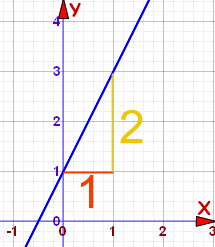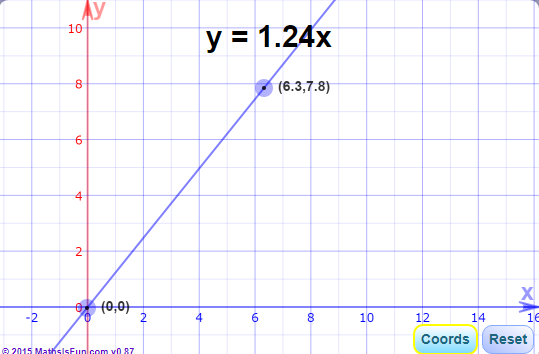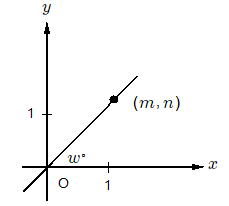# Line \bold{\LARGE{y = mx}}

## What does it stand for?y = how far up

x = how far along

m = Slope or Gradient (how steep the line is)

b = the y Intercept (where the line crosses the y axis)

## How do you find "m" and "b"?

b is easy: just see where the line crosses the y-axis.

m (the Slope) needs some calculation:

\bold{m = \dfrac{\text{Change in} \space y\text{-axis}}{\text{Change in} \space x\text{-axis}}}## Knowing this we can work out the equation of a straight line:

### Example 1m = \dfrac{2}{1} = 2

b = 1 (where the line crosses the y-Axis)

So: y = 2x + 1

### Example 2m = \dfrac{-3}{1} = -3

b = 0

This gives us y = -3x + 0

We do not need the zero!

So: y = -3x

## Rise and RunSometimes the words "rise" and "run" are used.

\bold{Rise} is how far up

\bold{Run} is how far along

And so the slope "m" is:

\bold{m = \dfrac{rise}{run}}

You might find that easier to remember

## Important Factif the |slope| (absolute value) of the line is greater than 1, then the angle of the line with x-axis greater than 45\degree

if the angle of the line with x-axis greater than 45\degree, then the |slope| (absolute value) of the line is greater than 1## Practice Questionw \gt 45
 Quantity A Quantity B m + n 2m

A. The quantity in Column A is greater

B. The quantity in Column B is greater

C. The two quantities are equal

D. The relationship cannot be determined from the information given

The equation for a line is:

\bold{y = kx + b}

where m is the slope and b is the y-intercept. Here, we are told that the line passes through the point \space (0,0) and point \space (m,n).

for m and n, we find that: m \gt 1 and n \gt 1, it is obvious that m \not = 0 and n \not = 0

Passing point \space (0,0) means that the y-intercept is 0. So the formula for line k is:

\bold{y = kx}

In order to compare A and B, let's try to subsract A by B:

m + n - 2m = n - m

So the question is to compare m and n.w \gt 45\degree \to n \gt m, so